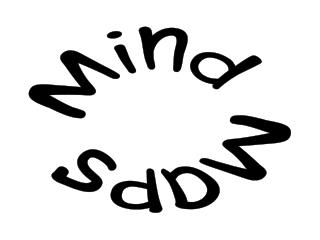DownloadDownload PresentationMind Maps

# Mind Maps

Télécharger la présentation## Mind Maps

- - - - - - - - - - - - - - - - - - - - - - - - - - - E N D - - - - - - - - - - - - - - - - - - - - - - - - - - -
##### Presentation Transcript

1. Mind Maps

2. A template for making your own mind map

3. An example of a mind map

4. m = mass Dh Work x F P= DW but DW = FDx so P = FDx or P = Fv Dt Dt ‘unstopability’ units kgms-1 what happens when things collide? Ans: r = mn Conservation of r: total r is the same before and after Power=rate of en transfer Momentum r . Total en before = Total en after Change in r = final r - initial r Dr = r1 + r2 2 m/s 1 m/s Conservation of Energy 0.3 kg Before 0.3 kg After g = 9.8N/kg = strength of gravity = force that a 1kg mass feels at the surface of the Earth Dynamics and Mechanical Energy Units C and D 1.10 - 1.16 Force = Dr/t N2L rate of change of momentum F = mv - mu = m(v - u) = ma t t iff m is constant Dgpe = mgDh units Ns F Impulse Impulse Close to surface DW = FDx SF= ma t I = Ft = mv - mu = Dr BIG F small t = small F BIG t = Dr KE = 1mv2 DW when F not // to x Expt F µ 1/m F µ a v 3F v 1m 2F 2m 1F 3m Energy transferred = Work done v t t

5. Topics in GCSE

6. D1 E K Electricity and Magnetism A B C J Forces and Motion Physics GCSE G L M Investigations 20% Waves H N D2 The Earth and Beyond Radioactivity F I Energy Sources and Energy Transfer

7. Topics in AS/A2

8. U1 Mechanics and Radioactivity U2 Electricity and Thermal Physics AS is U1 to 3 A2 is U4 to 6 Physics AS/A2 U3 Topics (e.g. Astrophysics) Practical Work U4 Waves and our Universe U6 Synthesis U5 Fields and Forces

9. A template for making a mind map on the computer (Careful, these can take a lot of time!)

10. . . . . . . . . . . . . . . . . . . . . . . . . . . . . . . . . . .